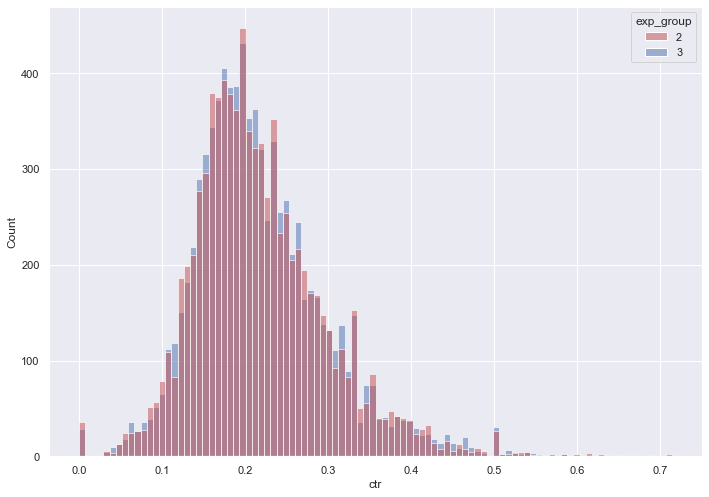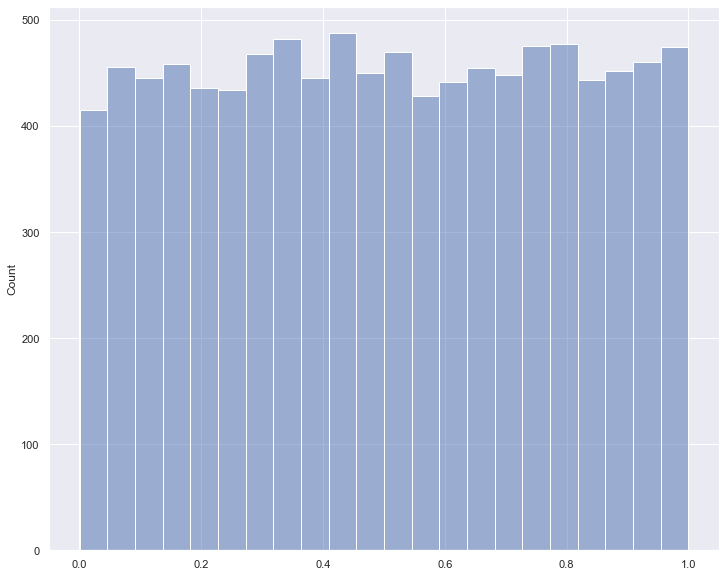I continue my series of publications on data analysis. This time we will look at AB testing. But initially, let's start with AA testing

When conducting an AA test, it would be good to make sure that our splitting system works correctly, and the key metric does not differ between groups. If the splitting system works correctly, then statistically significant differences between the two groups would occur only as a result of an accidental false positive. For example, if we accept the null hypothesis, provided that p_value < 0.05, then only in about 5% of cases we would have statistically significant differences between the groups.

To conduct the test, we will repeatedly extract subsamples with repetitions from our data and conduct a t-test, and at the end we will see in what percentage of cases we managed to reject the null hypothesis.

```import swifter
import hashlib
import pandas as pd
import pandahouse as ph
import numpy as np
import matplotlib.pyplot as plt
import seaborn as sns
from scipy import stats
```

Connecting to a data base in which a division into five groups has already been created earlier

```connection = {
'host': 'https://clickhouse.lab.karpov.courses',
'user': 'student',
'database': 'simulator_20220620'
}
```

We get only groups 2 and 3 from the database

```q = """
SELECT toDate(time) as Data, countIf(action='like') as Likes, countIf(action='view') as Vievs
FROM simulator_20220620.feed_actions
GROUP BY toDate(time)
"""
```
```df = ph.read_clickhouse(q, connection=connection)
```
```df.groupby('exp_group').count()
```
user_id likes views ctr
exp_group
2 8480 8480 8480 8480
3 8569 8569 8569 8569
```sns.set(rc={'figure.figsize':(11.7,8.27)})

groups = sns.histplot(data = df,
x='ctr',
hue='exp_group',
palette = ['r', 'b'],
alpha=0.5,
kde=False)
```We are launching 10,000 T-tests

```p_values = []
for _ in range(10000):
group_a = np.random.choice(df[df['exp_group'] == 2]['ctr'], size=500, replace=False)
group_b = np.random.choice(df[df['exp_group'] == 3]['ctr'], size=500, replace=False)
ttest = stats.ttest_ind(group_a,
group_b,
equal_var=False)§
p_values.append(ttest)
```

Now, in order to test our AA test, we will perform the following steps:

1. Build a histogram of the distribution of the resulting 10000 p-values.

```p_values = np.asarray(p_values)
```
```sns.set(rc={'figure.figsize':(12,10)})

groups = sns.histplot(data = p_values,
alpha=0.5,
kde=False)
```2. Calculate what percentage of p values turned out to be less than or equal to 0.05

```pv_low = (p_values <= 0.05).sum()
```

3. Write a conclusion based on the AA-test, whether our splitting system works correctly.

```print(f'p-values less than 0.05 in {str(pv_low / 10000 * 100)}% of cases. Means that in so many cases groups 2 and 3 have statistically significant differences. This is less than 5%, which means that the groups in the AA test are not statistically different.')
```
```p-values less than 0.05 in 4.6% of cases. Means that in so many cases groups 2 and 3 have statistically significant differences. This is less than 5%, which means that the groups in the AA test are not statistically different.
```